MATLAB Function Reference Go to function: Search    Help Desk

Syntax

```FX = gradient(F)
```

Definition

The gradient of a function of two variables, F(x,y), is defined as: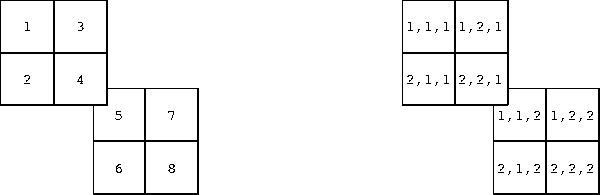and can be thought of as a collection of vectors pointing in the direction of increasing values of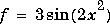In MATLAB, numerical gradients (differences) can be computed for functions with any number of variables. For a function of N variables, F(x,y,z,...),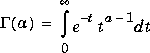Description

```FX = gradient(F) ``` where `F` is a vector returns the one-dimensional numerical gradient of `F`. `FX` corresponds to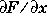, the differences in the x direction.

```[FX,FY] = gradient(F) ``` where `F` is a matrix returns the x and y components of the two-dimensional numerical gradient. `FX` corresponds to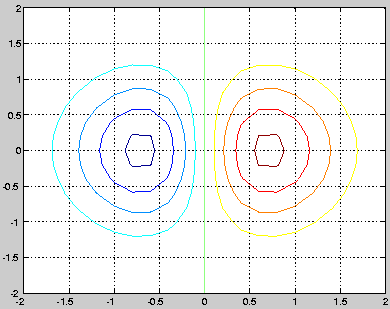, the differences in the `x` (column) direction. `FY` corresponds to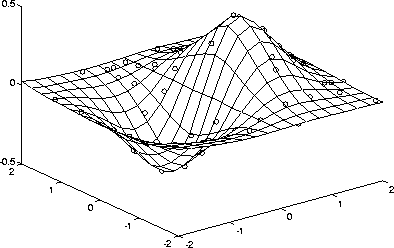, the differences in the y (row) direction. The spacing between points in each direction is assumed to be one.

```[FX,FY,FZ,...] = gradient(F) ``` where `F` has `N dimensions` returns the `N` components of the gradient of `F`.

There are two ways to control the spacing between values in `F`:

A single spacing value, `h`, specifies the spacing between points in every direction.

`N` spacing values (`h1,h2,...`) specify the spacing for each dimension of `F`. Scalar spacing parameters specify a constant spacing for each dimension. Vector parameters specify the coordinates of the values along corresponding dimensions of `F`. In this case, the length of the vector must match the size of the corresponding dimension.

```[...] = gradient(F,h) ``` where `h` is a scalar uses `h` as the spacing between points in each direction.

```[...] = gradient(F,h1,h2,...) ``` with `N` spacing parameters specifies the spacing for each dimension of `F`.

Examples

The statements

```v = -2:0.2:2;
[x,y] = meshgrid(v);
z = x .* exp(-x.^2 - y.^2);
contour(v,v,z), hold on, quiver(px,py), hold off
```
produce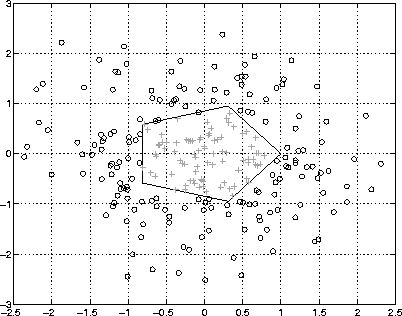Given,

```F(:,:,1) = magic(3); F(:,:,2) = pascal(3);
`gradient(F)` takes `dx` = `dy` = `dz` = `1`.
`[PX,PY,PZ] = gradient(F,0.2,0.1,0.2)` takes `dx = 0.2`, `dy = 0.1`, and
`dz = 0.2`.
```

`del2``        `Discrete Laplacian
`diff``        `Differences and approximate derivatives